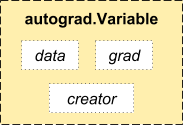# 自动求导: 自动微分

PyTorch 中所有神经网络的核心是 autograd 自动求导包. 我们先来简单介绍一下, 然后我们会去训练我们的第一个神经网络.

autograd 自动求导包针对张量上的所有操作都提供了自动微分操作. 这是一个逐个运行的框架, 这意味着您的反向传播是由您的代码如何运行来定义的, 每个单一的迭代都可以不一样.

## Variable（变量）

autograd.Variable 是包的核心类. 它包装了张量, 并且支持几乎所有的操作. 一旦你完成了你的计算, 你就可以调用 .backward() 方法, 然后所有的梯度计算会自动进行.Variable

VariableFunction 是相互联系的, 并且它们构建了一个非循环的图, 编码了一个完整的计算历史信息. 每一个 variable（变量）都有一个 .grad_fn 属性, 它引用了一个已经创建了 VariableFunction （除了用户创建的 Variable 之外 - 它们的 grad_fnNone ）.

import torch


x = Variable(torch.ones(2, 2), requires_grad = True)
print(x)


variable（变量）的操作:

y = x + 2
print(y)


y 由操作创建,所以它有 grad_fn 属性.

print(y.grad_fn)


y 的更多操作

z = y * y * 3
out = z.mean()

print(z, out)


## 梯度

out.backward()


print(x.grad)


x = torch.randn(3)
x = Variable(x, requires_grad = True)

y = x * 2
while y.data.norm() < 1000:
y = y * 2

print(y)

gradients = torch.FloatTensor([0.1, 1.0, 0.0001])

VariableFunction 的文档请参阅 http://pytorch.apachecn.org/cn/docs/0.3.0/autograd.html